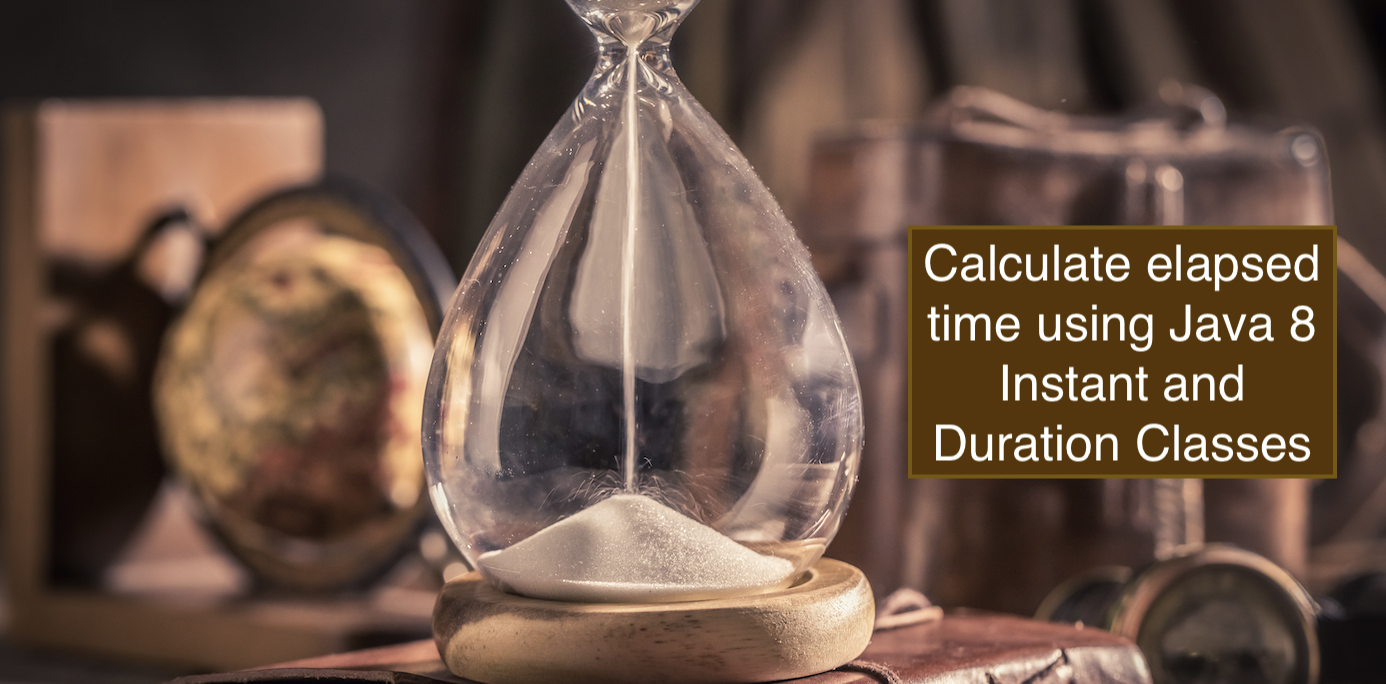# Best way to calculate elapsed time in Java using Java 8 Duration & Instant Class with Nanoseconds precisionIf you are using Java 8 and above then you should definitely try out using java.time API for dealing with date and time. In order to find how much time has elapsed between an event A (start) and even B (end), you should make use of Instant and the Duration class to get the best results,

Example:

First we create an object that holds instant in time before we start,

``Instant eventA = Instant.now() //Capture the instant in time before we start ``

Now we have our logic that we want to test how much time it takes to execute (most of us will like to test algorithms like sorting)

``````for (int i=0;i<10;i++) {
}``````

First we create an object that holds instant in time before we start,

``Instant eventB = Instant.now() //Instant in time after completion our logic execution.``

Now let's calculate the difference between these two events,

``Duration timeElapsed = Duration.between(eventA,eventB);``

Sample outputs of multiple runs,

PT10.037126S
PT10.038047S
PT10.036216S
PT10.031141S
PT10.032412S
PT10.032311S

Complete Code:
``````package org.code2care;

import java.time.Duration;
import java.time.Instant;

/**
* Java Programs by Code2care.org
*/